Skip to content
Related Articles
Excel – Working with DATE Functions With Examples
• Difficulty Level : Easy
• Last Updated : 20 May, 2021

The DATE function is used to calculate dates in Excel. Excel provides different functions to work with dates & times such as TODAY, NOW, WEEKDAY, EOMONTH, etc. which we will discuss here with examples.

The purpose of discussing DATE functions in Excel is to help different people to perform more complex and challenging tasks by combining several functions within one formula.

### DATE function

It will return the date in serial number based on the year, month, or day value as provided.

```Syntax:DATE(year,month,day)

Arguments:

1.Year:-This argument includes 1 to 4 digit value. Excel understand this year argument
according to the date system of the local computer which we use.
For example-Excel windows uses 1900 date system by default which
means DATE(21,2,6) gives result as 06-02-1921.
2.Month:-This argument include positive or negative integer which represents the month
of year from January to Decemeber.
3.Day:-This argument also include positive or negative integer representing day of the month
from 1 to 31.```

Example 1: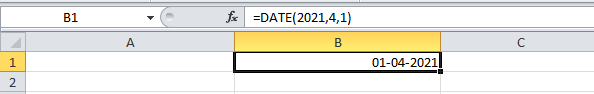Example 2: It will return on the first day of the current year & month.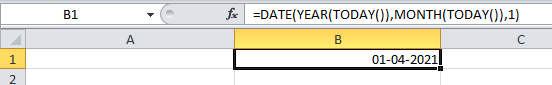Example 3: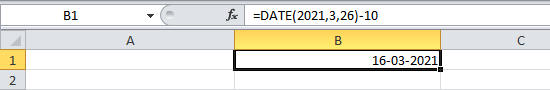### TODAY function

The TODAY() function name suggests it will return today’s date, and it has no arguments.

`Syntax: TODAY()`

Example1: Here we will print the current date and also add 10 days to the current date.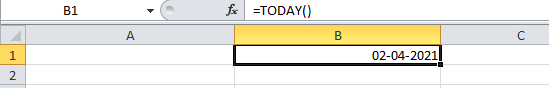Example 2: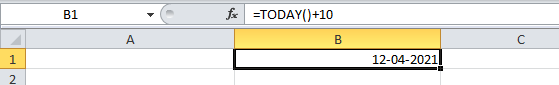### NOW function

This function returns the current date as well as time & doesn’t have any arguments.

`Syntax: NOW()`

Example: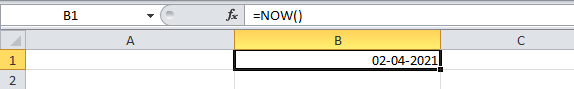### DATEVALUE function

It converts the date in text format to a serial number which can be represented as a date.

```Syntax: DATEVALUE(date_text)

Arguments:
1. date_text:-This argument is a text that represents the date in Excel date format.```

Example: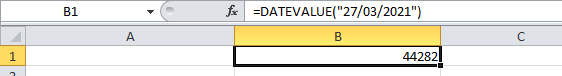### TEXT function

It converts any numeric value not only dates to a text string. Through this function, we can change the date to text strings in a variety of formats.

```Syntax: TEXT(value,format_text)

Arguments:
1. value: The value that is to be converted.
2. format_text: The format in which you want to output the date value.```

These are the different formats used in the TEXT function to change dates to text strings.

Example 1: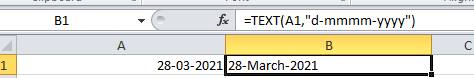Example 2: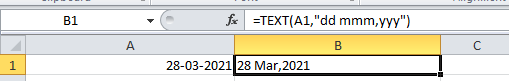Example 3: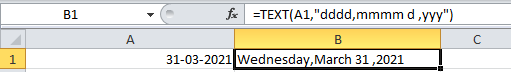### DAY function

It returns the day of a month i.e. integer from 1 to 31.

```Syntax: DAY(serial_number)

Arguments:
1. serial_number: This value represents the day of the month you want to find.
E.g: 5th day of june```

Example 1: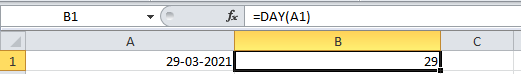Example 2: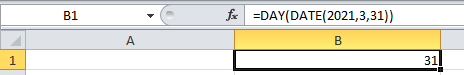The DAY(TODAY()) function returns the day of today’s date as shown below: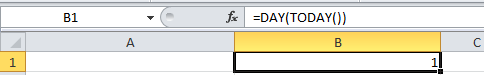### MONTH function

This function returns the month of the given date as an integer from 1 to 12 (January to December).

```Syntax: MONTH(serial_number)

Arguments:
1. serial_number: This value represents the date for which you want to find the month.```

Example: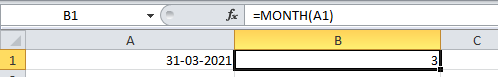The MONTH(TODAY())  function returns the month of today’s date.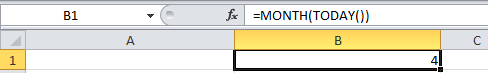### YEAR function

It returns the year of a specified date.

```Syntax: YEAR(serial_number)

Arguments:
1. serial_number: The date to be specified.```

Example 1: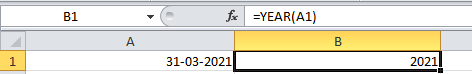Example 2: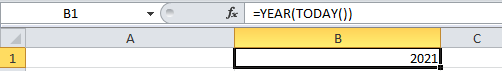Example 3: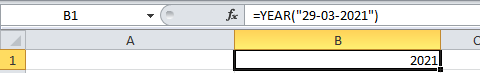### EOMONTH function

This function returns the last day of the month after adding a specified number of months to a given date.

```Syntax: EOMONTH(start_date,months)

Arguments:
1. start_date: In this argument, the date should be written in date format, not in the text.
2. months: In this argument, if a positive integer is given then corresponding months can be
added to the start date & if a negative integer is given then corresponding months
can be subtracted to the start date.```

Example 1: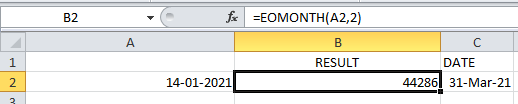Example 2: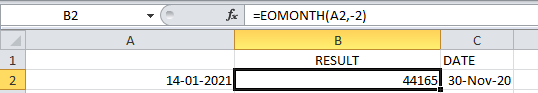Example 3: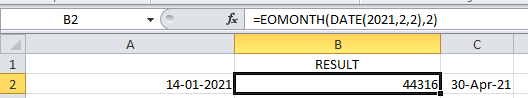The EOMONTH(TODAY(),0) function returns the last day of the current month.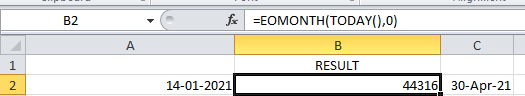### WEEKDAY function

This function returns the day of the week as a number from 1 to 7(Sunday to  Saturday) according to the specified date.

```Syntax: WEEKDAY(serial_number,return_type)

Arguments:
1. serial_number: It can be a date or the cell that contains the date.
2. return_type: It is optional as it specifies which day should be considered
as the first day of week.```

NOTE:1st day of the week is by default Sunday.

Example 1: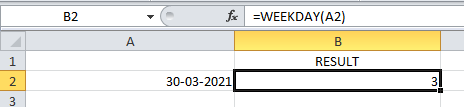Example 2: In the below example, 2 is given as return_type i.e. Monday is referred to as the first day of the week.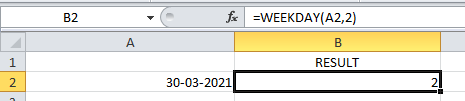Example 3:

Here the day of todays(01-04-2021) date is the result & the default value (Sunday) is considered here because no return_type is given.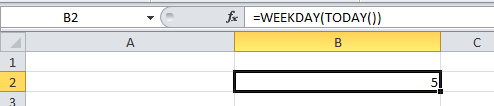### DATEDIF function

This function calculates the difference between two dates in days, months, or years.

For calculating the difference b/w dates which time interval should be used depends on the letter which we specify in our last argument i.e. at the unit.

```Syntax: DATEDIF(start_date,end_date,unit)

Arguments:
1. start_date: The start date for evaluating the difference.
2. end_date: The end Date for evaluating the difference.```

Example 1: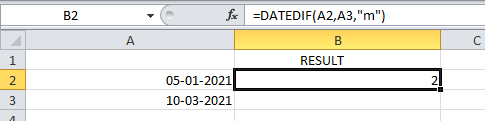Example 2: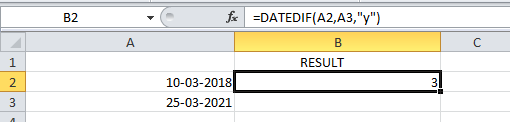Example 3: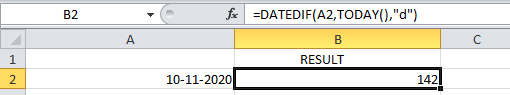Here “m”,”y”,”d” means month, year & date. In the first example, the difference between dates is calculated by months, second by year & third by date.

### WEEKNUM function

It returns the week number based on the specified date i.e. from 1 to 52 weeks of the year.

```Syntax: WEEKNUM(serial_number,firstday_ofweek)

Arguments:
1. serial_number:This is the date for which we want the week number.
2. firstday_ofweek: This is optional arguments that specify which numbering
system should be considered & which day of the week can be
treated as start of the week,Default(omitted) is 1.
The table below is the parameters that can be given in
firstday_ofweek arguments.```

First Day of the Week Start Table

Example 1: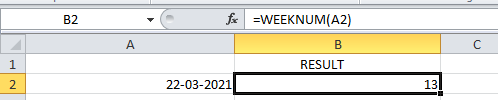Example 2: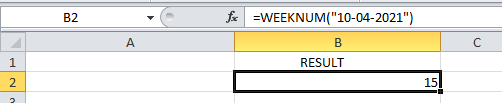Example 3:

In the below example,21 is given as the second argument that means Monday is taken as the first day of the week & in the above example, the result shown is 15 but by taking 21 as the first_dayofweek means Monday as the first day the result is 14.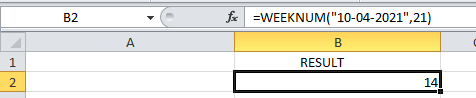### EDATE function

This function adds or subtracts the specified month to a given date.

```Syntax: EDATE(start_date,months)

Arguments:
1. start_date: This is an initial date on which the months are added or subtracted.
2. months: This is the number of months which is to be added or subtracted in the specified date.```

Example 1: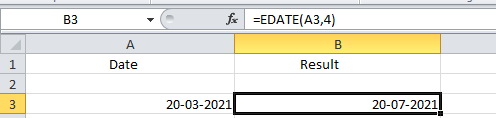Example 2: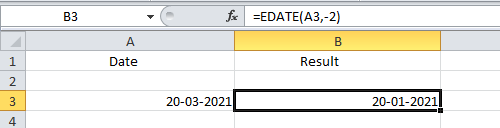Example 3: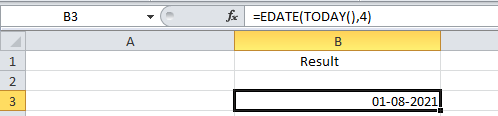### YEARFRAC function

This function returns the fraction of the year which represents the number of whole days between the start & end date.

```Syntax: YEARFRAC(start_date,end_date,[basis])

Arguments:
1. start_date: This is the start date in the serial number.
2. end_date: This is the end date in the serial number.
3. basis: This is the optional argument that specifies the day count method.```

Example 1: Using someday count methods.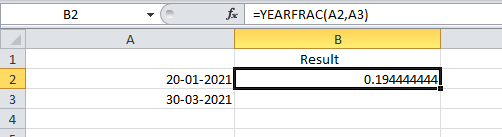Example 2: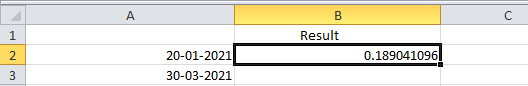Example 3: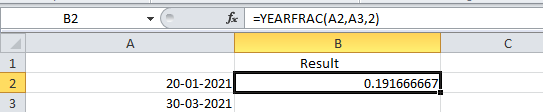### WORKDAY function

This function helps if we exactly know how many working days we have & want to find out the date when the number of working will skip. This function always includes working days & excludes weekend days.

```Syntax: WORKDAY(start_date,days,holidays)

Arguments:
1. start_date: This argument is the date from which the counting of weekdays begins.
Excel doesn't include start_date as a working day.
2. days: This is the number of working days.
3. holidays: This is an optional argument. If the days mentioned include any
holidays then we need to make a list of holidays separately for
this and mention it here.```

Example 1: 28 workdays from the start date, excluding holidays.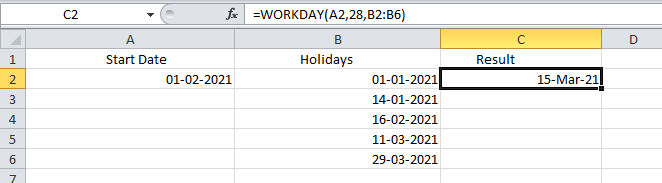Example 2: 28 workdays before the start date, excluding holidays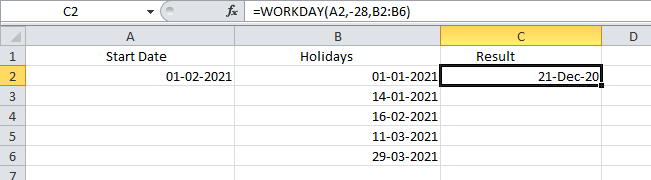Example 3: 28 workdays from the start date, no holidays.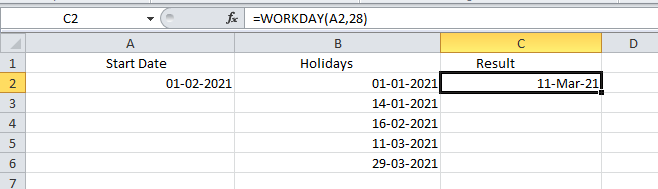Example 4: 28 workdays from today’s date, no holidays.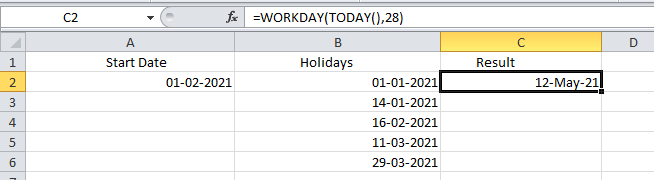### WORKDAY.INTL function

This is a modification of the WORKDAY function as it provides a custom weekend parameter which distinguishes this from the WORKDAY function.

```Syntax: WORKDAY.INTL(start_date,days,[weekends],holidays)

Arguments:
1. start_date: This argument is the date from which the counting of weekdays begins.
Excel doesn't include start_date as a working day.
2. days: This is the number of working days.
3. holidays: This is an optional argument. If the days mentioned include any
holidays then we need to make a list of holidays separately for
this and mention it here.
4. weekends: Through this argument we can specify which days of the week to be treated
as non working days either by weekend number or specific character string.```

### Weekend number:

If this weekend argument is blank in this function then it will automatically take the combination of Saturday & Sunday.

For instance:

• “0000011”-Saturday & Sunday are weekends(non-working days)
• “1000010”-Monday & Saturday are weekends(non-working days)

Example 1: 30 days from the start date, excluding holidays & Sunday, Monday as weekends(by giving weekend number 2 as arguments).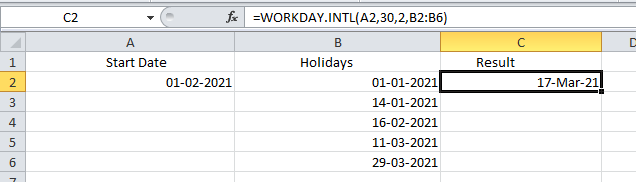Example 2: 30 days from the start date, excluding holidays & Sunday, Monday as weekends(by giving weekend string “1000001” as arguments).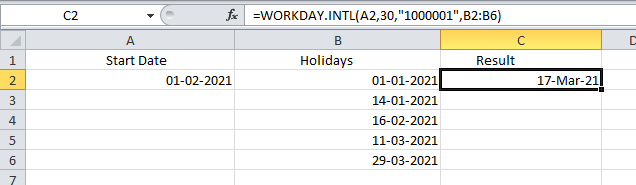Example 3: 20 days from the start date, no holidays & Monday, Saturday as weekends( by giving weekend string “1000010” as arguments).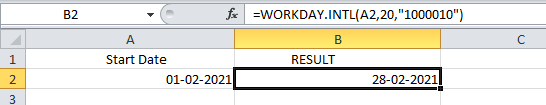### NETWORKDAYS function

This function returns the number of working days between two dates excluding weekends & holidays is as optional arguments.

```Syntax: NETWORKDAYS(start_date,end_date,holidays)

Arguments:
1. start_date: The initial date to start evaluation.
2. end_date: The last date to end the evaluation.
4. holidays: Used to specify holidays.   ```

Example 1: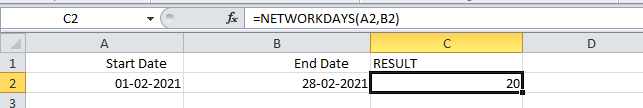Example 2: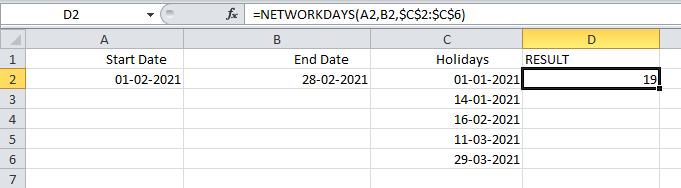### NETWORKDAYS.INTL function

This function also returns the number of working days between two dates but provides additional argument weekend to specify which days should be counted as weekend days.

The structure of the weekend argument is the same as for WORKDAY.INTL i.e. we can use either weekend number or character string.

```Syntax: NETWORKDAYS.INTL(start_date,end_date,[weekend],holidays)

Arguments:
1. start_date: The initial date to start evaluation.
2. end_date: The last date to end the evaluation.
3. weekend: Use to specify the weekends.
4. holidays: Used to specify holidays.  ```

Example 1: Here weekend argument is given in form of a number.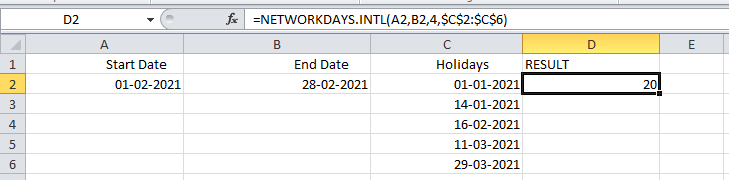Example 2: Here weekend argument is given in form of a character string of 0’s & 1’s.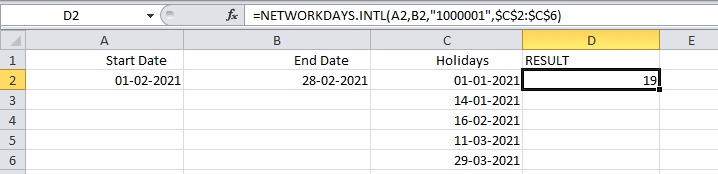My Personal Notes arrow_drop_up